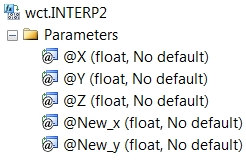# SQL Server 2-dimensional linear interpolation function

INTERP2

Updated: 30 September 2013

Use the aggregate function INTERP2 to interpolate on a 2-dimensional grid. INTERP2 expects the grid to be represented in 3rd normal form. INTERP2 is not sensitive to the order of the input.

The 2-dimensional or bilinear interpolation calculation can be thought of as a series of linear interpolation calculations using the closest combination of x- and y-values on the 2-dimensional grid. For example, let's look at the following table which shows the number of days in the future as the x-values, the strike price of an option as the y-values, and which contains the volatility associated with the date and the strike price.

SELECT x,y,z
INTO #v
FROM (VALUES
(30,70,0.22),
(30,80,0.2),
(30,90,0.18),
(30,100,0.16),
(30,110,0.175),
(30,120,0.19),
(30,130,0.205),
(90,70,0.23),
(90,80,0.21),
(90,90,0.19),
(90,100,0.17),
(90,110,0.185),
(90,120,0.2),
(90,130,0.215),
(182,70,0.26),
(182,80,0.24),
(182,90,0.22),
(182,100,0.2),
(182,110,0.215),
(182,120,0.23),
(182,130,0.245),
(270,70,0.25),
(270,80,0.23),
(270,90,0.21),
(270,100,0.19),
(270,110,0.205),
(270,120,0.22),
(270,130,0.235),
(365,70,0.245),
(365,80,0.225),
(365,90,0.205),
(365,100,0.185),
(365,110,0.2),
(365,120,0.215),
(365,130,0.23)
)n(x,y,z)

If we want to interpolate the volatility for 63 days with a strike price of 87, we can quickly see that the surrounding points would look like this.

 80 90 30 0.2 0.18 90 0.21 0.19

It is then a very straightforward to see that the desired result can be thought of as three linear interpolation calculations, as demonstrated by the following SQL.

SELECT wct.INTERP(y, z, 87) as INTERP2
FROM (
SELECT y, wct.INTERP(x,z,63) as z
FROM #v
WHERE y = (SELECT MAX(y) FROM #v WHERE y <= 87)
GROUP BY y
UNION
SELECT y, wct.INTERP(x,z,63)
FROM #v
WHERE y = (SELECT MIN(y) FROM #v WHERE y > 87)
GROUP BY y
)m

Producing the result of 0.1915
SyntaxArguments
@X
The value of the co-ordinate along the x-axis. @X must be of a type float or of a type that implicitly converts to float.
@Y
The value of the co-ordinate along the y-axis. @Y must be of a type float or of a type that implicitly converts to float.
@Z
The value contain at (@X,@Y). @Z must be of a type float or of a type that implicitly converts to float.
@New_x
the new x-value used by INTERP2 to calculate the interpolated z-value. @New_x must be of a type float or of a type that implicitly converts to float.
@New_y
the new y-value used by INTERP2 to calculate the interpolated z-value. @New_y must be of a type float or of a type that implicitly converts to float.
Return Types
float
Remarks
·         For data not in 3rd normal form, consider using the BILINEARINTERP function.
·         If @New_x is less than the smallest x-value or greater than the largest x-value in the array, an error will be returned.
·         If @New_x < MIN(@X) or @New_x > MAX(@X) then a NULL will be returned.
·         If @New_y < MIN(@Y) or @New_y > MAX(@Y) then a NULL will be returned.
·         If the @New_x and @New_y are bound by a grid containing a NULL then a NULL will be returned.
·         @New_x must remain invariant for the GROUP.
·         @New_y must remain invariant for the GROUP.
·         Available in XLeratorDB / math 2008 only
Examples
SELECT wct.INTERP2(x,y,z,63,87) as INTERP2
FROM (VALUES
(30,70,0.22),
(30,80,0.2),
(30,90,0.18),
(30,100,0.16),
(30,110,0.175),
(30,120,0.19),
(30,130,0.205),
(90,70,0.23),
(90,80,0.21),
(90,90,0.19),
(90,100,0.17),
(90,110,0.185),
(90,120,0.2),
(90,130,0.215),
(182,70,0.26),
(182,80,0.24),
(182,90,0.22),
(182,100,0.2),
(182,110,0.215),
(182,120,0.23),
(182,130,0.245),
(270,70,0.25),
(270,80,0.23),
(270,90,0.21),
(270,100,0.19),
(270,110,0.205),
(270,120,0.22),
(270,130,0.235),
(365,70,0.245),
(365,80,0.225),
(365,90,0.205),
(365,100,0.185),
(365,110,0.2),
(365,120,0.215),
(365,130,0.23)
)n(x,y,z)

This produces the following result.
INTERP2
----------------------
0.1915

### SupportCopyright 2008-2022 Westclintech LLC         Privacy Policy        Terms of Service